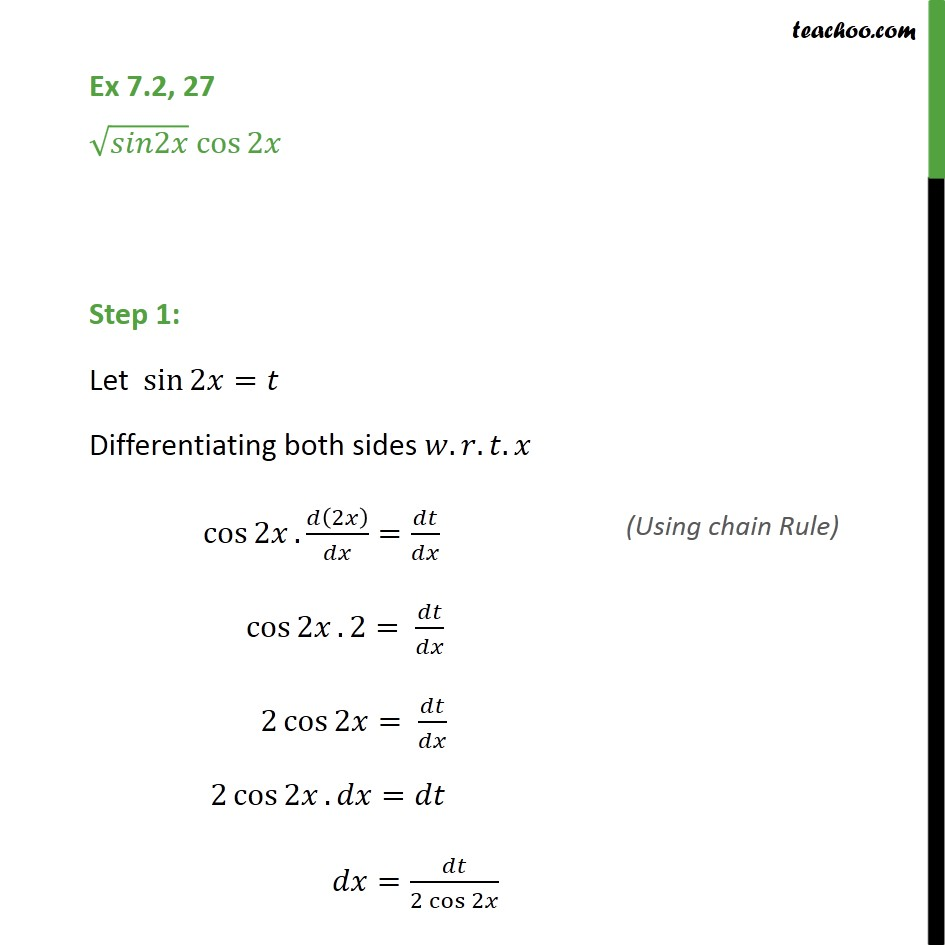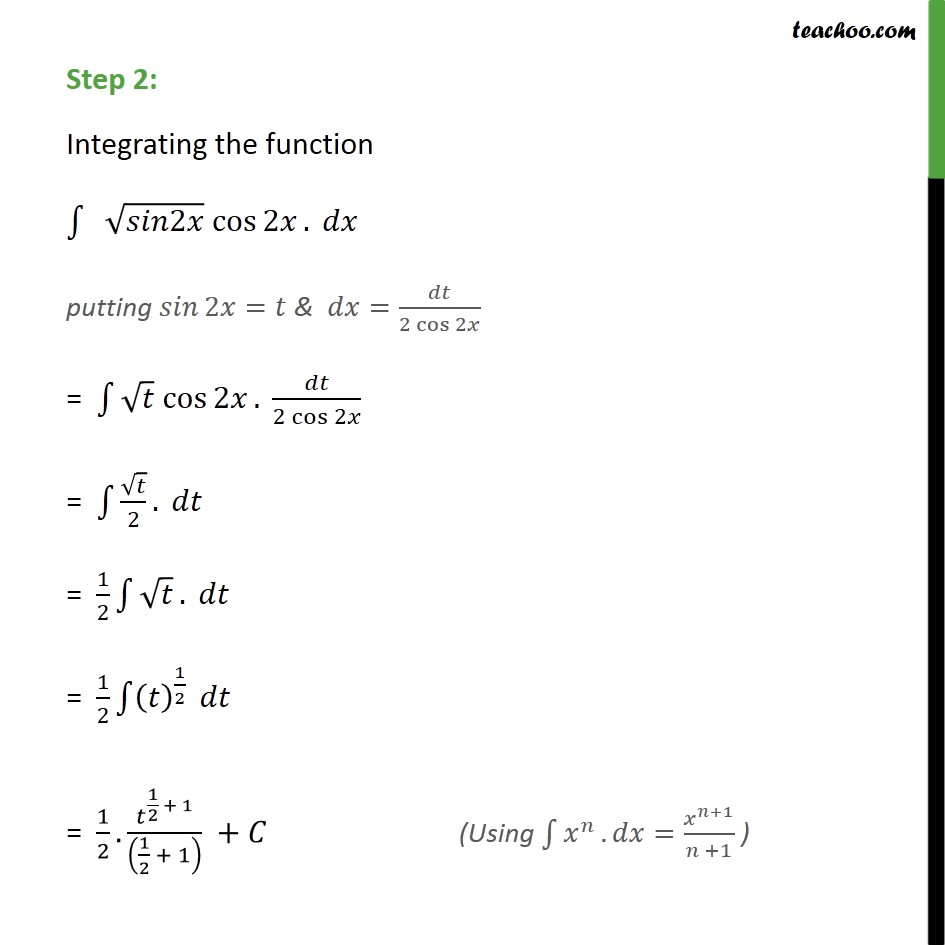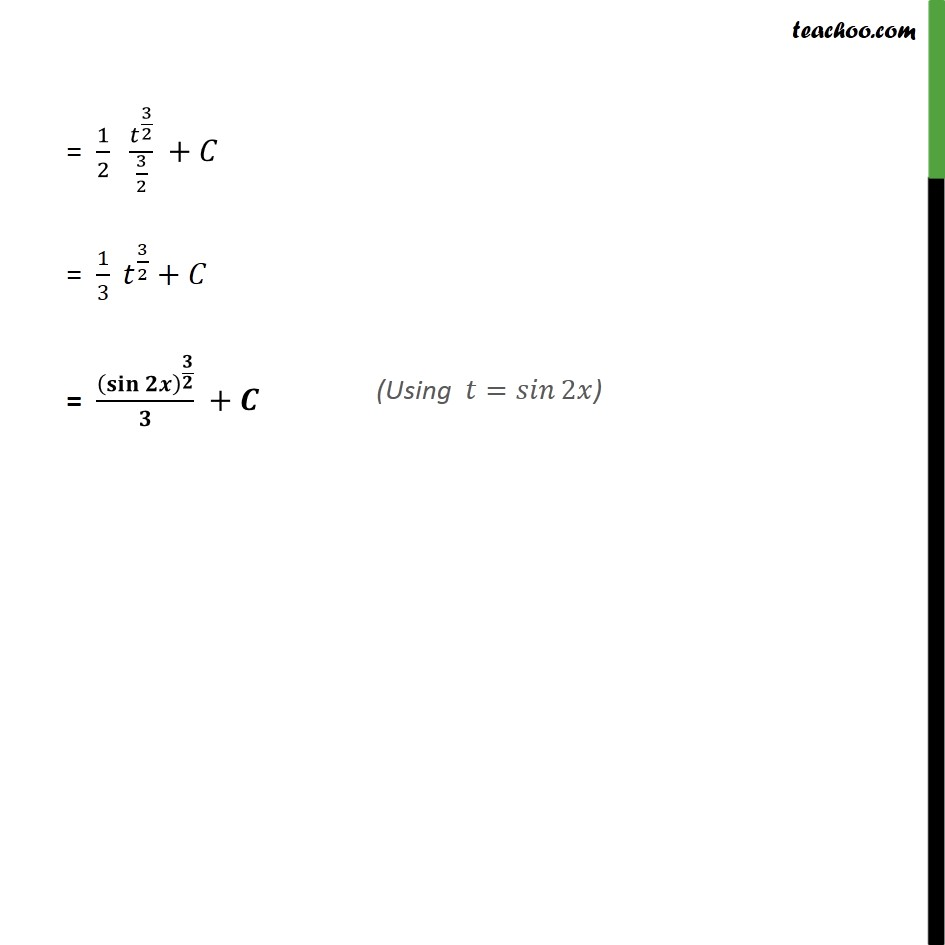1. Chapter 7 Class 12 Integrals (Term 2)
2. Concept wise
3. Integration by substitution - Trignometric - Normal

Transcript

Ex 7.2, 27 2 cos 2 Step 1: Let sin 2 = Differentiating both sides . . . cos 2 . 2 = cos 2 . 2= 2 cos 2 = 2 cos 2 . = = 2 cos 2 Step 2: Integrating the function 2 cos 2 . putting 2 = & = 2 cos 2 = cos 2 . 2 cos 2 = 2 . = 1 2 . = 1 2 1 2 = 1 2 . 1 2 + 1 1 2 + 1 + = 1 2 3 2 3 2 + = 1 3 3 2 + = +